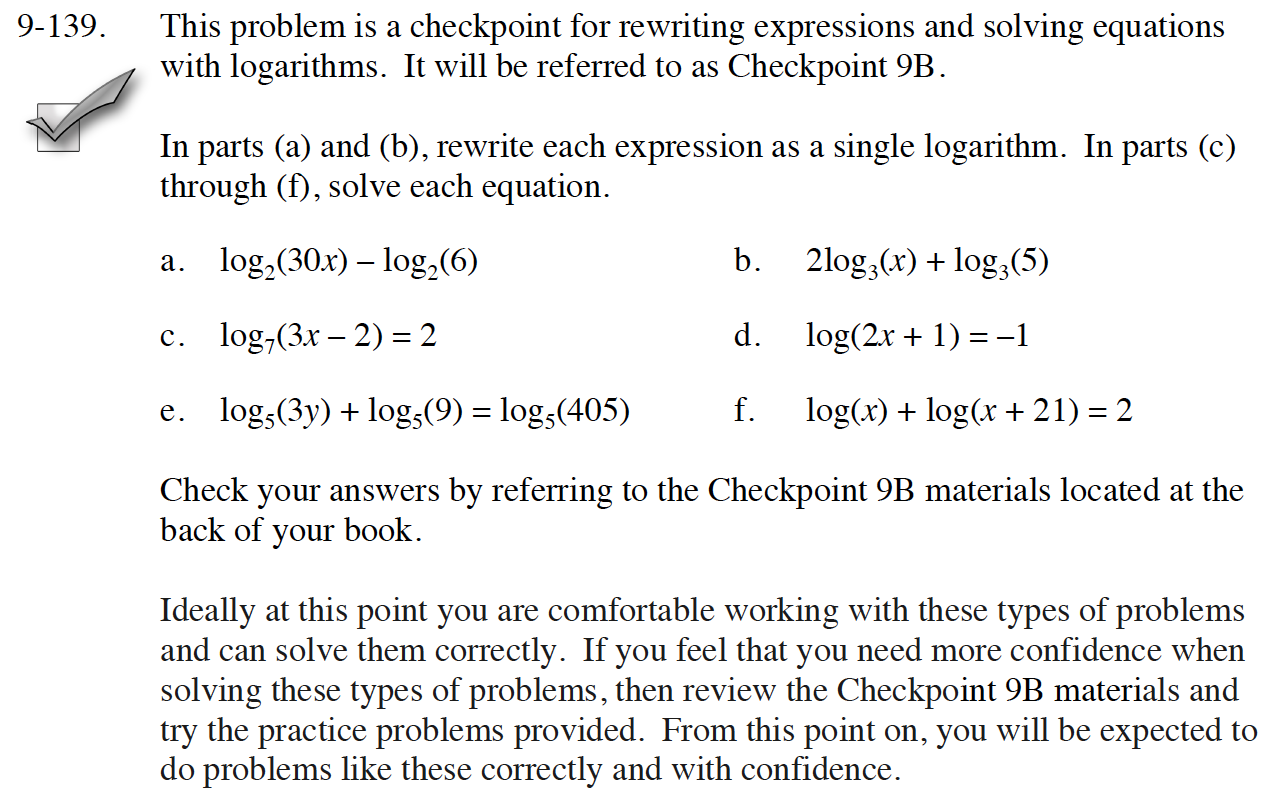### Home > INT3 > Chapter Ch9 > Lesson 9.2.4 > Problem9-139

9-139.
1.This problem is a checkpoint for rewriting expressions and solving equations with logarithms. It will be referred to as Checkpoint 9B.

In parts (a) and (b), rewrite each expression as a single logarithm. In parts (c) through (f), solve each equation. Homework Help ✎

1. log2(30x) – log2(6)

2. 2log3(x) + log3(5)

3. log7(3x – 2) = 2

4. log(2x + 1) = –1

5. log5(3y) + log5(9) = log5(405)

6. log(x) + log(x + 21) = 2

2. Check your answers by referring to the Checkpoint 9B materials located at the back of your book.

Ideally at this point you are comfortable working with these types of problems and can solve them correctly. If you feel that you need more confidence when solving these types of problems, then review the Checkpoint 9B materials and try the practice problems provided. From this point on, you will be expected to do problems like these correctly and with confidence.Answers and extra practice are located in the back of your printed textbook or in the Reference Tab of your eBook. If you have an eBook for Int3, login and then click the following link: Checkpoint 9B Answers and Practice